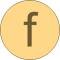# normalizeWithAssert

Return normalized vector such that length = 1 (trigger an assert for zero vector)# Information

This information is part of the Modelica Standard Library maintained by the Modelica Association.

#### Syntax

```Vectors.normalizeWithAssert(v);
```

#### Description

The function call "`Vectors.normalizeWithAssert(v)`" returns the unit vector "`v/sqrt(v*v)`" of vector v. If vector v is a zero vector, an assert is triggered.

Since the function has the "Inline" annotation, it is usually inlined and symbolic processing is applied.

#### Example

```  normalizeWithAssert({1,2,3});  // = {0.267, 0.534, 0.802}
normalizeWithAssert({0,0,0});  // error (an assert is triggered)
```